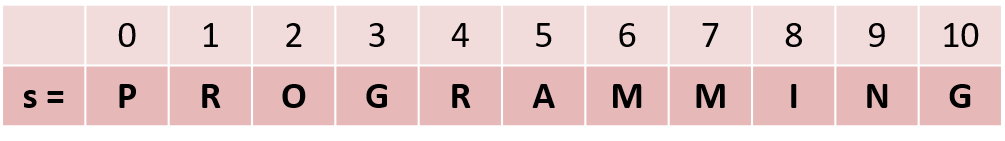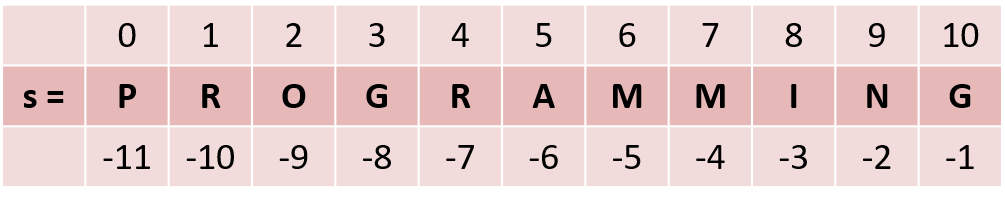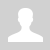Python. Strings (Строки)

11.2.2.3 apply functions and string processing methods
11.2.3.6 determine the difference between different data structures

Python. Strings (Строки)

## Strings. Indexing

We already saw the Set collection in the previous lesson, which can store more than one value. Today we are getting acquainted with the second collection - String. And although we have often used the string type, this is the first time we will consider a string as a collection of symbols.

String is a complex data type that stores a sequence of characters.

We get the string when we enclose characters in quotation marks, for example, "text", when we use input() when we use the conversion function str ().

We can already measure the length of a string using the len() function and determine the occurrence of one string within another using the in operation.

``````fixed_line = "programming"
print(len(fixed_line)) # print line length
word = input () # input string
print(word) # line output
number = 2020
line = str(number) # convert number to string
print(line)
print("program" in fixed_line) # checking the occurrence of one line in another``````

Unlike sets, which contain unordered elements, the sequence of characters in a string has its own order, and each character has its own address in the string - an index by which it can be accessed.You can refer to each character by its index. To do this, use the following entry:

s - refer to the character with index 1 in string s.

``````s = "programming"
print(s) # print the letter "r"
print(s + s + s) # output of the word "map"``````

Negative indexes

Unlike many languages, Python also uses negative indices.``````s = "programming"
print(s[-10]) # print the letter "r"
print(s[-5] + s[-6] + s[-11]) # output of the word "map"``````

When accessing an index that does not exist, we will get an error.

``````s = "programming"
print(s) # IndexError: string index out of range
# Index error: row index is out of range``````

If we want to correct one letter in a word, for example,

``````s = "pragramming"
s = "o" # TypeError: 'str' object does not support item assignment``````

The Python interpreter throws an error - it means that it is impossible to change a single character of the string, that is, the string is an immutable data type in Python.

## Iterating over the elements of a string.

Since a string is a collection that consists of character elements, we can iterate over each element of the string as we iterate over sets. To iterate over, we will use a for a loop.

``````s = "programming"
for item in s: # iterator item will iterate over each character of the string
print(item, end=" ") # output each character of the string separated by a space``````

String data structure features:

• Strings are an ordered data structure. Each element has its own index. Indexing starts from 0 (zero).
• Strings are an iterable data structure. You can iterate over the elements of strings in two ways, by characters, and by indices.
• Each element of the string is represented by one character.
• Strings are an immutable data structure. You cannot replace a character in a string without conversions.

Task. Determine how many vowels are in the line.

``````vowels = {"а", "е", "i", "o", "u"} # vowels set
line = "Remote education" # string
n = 0 # number of vowels
for i in line: # iterate over characters in line
if i in vowels: # is i a vowel
n += 1 # counting vowels
print(n) # output the result ``````

But, since the characters in the string are numbered, we have another way to iterate over all the elements in the string: iterate over all indices using the already familiar for i in range (...) construction.

``````vowels = "аеiou" # declare vowels string
line = "Remote education" # string
n = 0 # number of vowels
for i in range(len(line)): # enumeration by indices
if line[i] in vowels: # checking the i-th element in a vowel string
n += 1 # counting vowels
print(n) # output the result``````

Questions:

Exercises:

Категория: Algorithms | Добавил: bzfar77 (15.11.2021)
Просмотров: 388 | Теги: String, Iteration, symbol, Concatenation, Index | Рейтинг: 5.0/2
 Всего комментариев: 0